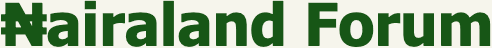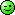#Welcome, Guest: Join Nairaland / LOGIN! / Trending / Recent / New
Stats: 2,865,262 members, 6,875,436 topics. Date: Sunday, 22 May 2022 at 05:46 PM

## Program To Add To Polynomials - Programming - Nairaland

 Program To Add To Polynomials by logic101: 10:39pm On Jul 25, 2011 Hi am a computer science student working on a particular question to perform certain functions with polynomials.I have two classes, my monomial class and my polynomial class. I am trying to add two polynomials but i keep gtting a null pointer exception in my add method.Can the house help , Fayimora u seem well infromed can you chip in .public class Polynimial { Monomial [] polynomial;; int degree; Scanner in= new Scanner(System.in); // int a is coefficient while int b is the exponent public Polynimial(int c){ polynomial= new Monomial[c+1]; } public static void main(String[] args) { Polynimial c= new Polynimial(1); c.setPolynomial(); Polynimial d = new Polynimial(1); d.setPolynomial(); System.out.println(c.plus(d)); } public void setPolynomial(){ int x=polynomial.length; int count=0; System.out.println("please enter in this particular order all the coefficient and exponent "; while(count 2x3 + 2x2 + 4x + 1 = 0So how do I key in the values? Also, you got some really bad names here that can cause a brain damage, lol Just kidding but your variable names are confusing, Re: Program To Add To Polynomials by logic101: 10:54pm On Jul 25, 2011 lol fayimora yup my variable names are u knwww, i would take that into consideration lol, u can key in the values in the main program which is in the polynomial class, Re: Program To Add To Polynomials by Fayimora(m): 10:56pm On Jul 25, 2011 No i mean during execution. You have this:What do i key in(use the example above), that instruction is not descriptive enough! Re: Program To Add To Polynomials by logic101: 11:04pm On Jul 25, 2011 oh alryt e.g lets say u want to add 5x^3 + 2X^2 + 2x +9 and 4x^3 + 2X^2+7x +5for first polynomial u wld entr the max exponent of each polynomial into its object reference, so it can be input in to ways frm exponent o which wld be coefficient, exponent90212253 Re: Program To Add To Polynomials by Fayimora(m): 11:08pm On Jul 25, 2011 Well the bad news is that you have more problems than you thought` public void setPolynomial(){ int x=polynomial.length; int count=0; System.out.println("Please enter in this particular order all the coefficient and exponent "; while(count < x){ int coefficient=in.nextInt(); int exponent=in.nextInt(); polynomial[exponent]=new Monomial(coefficient,exponent); count++; } }`Help me to understand why you have this:int x=polynomial.length; Re: Program To Add To Polynomials by logic101: 11:13pm On Jul 25, 2011 its used in the while loop as a condition to input the coefficientss so if the lenght of the polynomial is 6, it needs 6 coefficients starting frm index 0-5 Re: Program To Add To Polynomials by Fayimora(m): 11:18pm On Jul 25, 2011 But i dnt see where you check the length of the polynomial, Weird! Do you mind re-writing this code? They are easier ways to do this i guess. Do you have a requirement or you just have to implement in in anyway.If you dont have a requirement then the fastest way to do this is with a Map. Do you want to re-write it? Don't worr about not knowing how to use a map I would walk you through the whole process, but you would do the reasoning part.Reason why i want you to rewrite the code is because what you have here has a lot of redundancies and its very confusing(e.g you called a variable of type Monomial `polynomial`) Its just difficult to comprehend. Re: Program To Add To Polynomials by Fayimora(m): 4:22am On Jul 26, 2011 Hey sorry was baned by the spambot, Have a look at what i posted which is marked as spamRe: Program To Add To Polynomials by Nobody: 5:46pm On Aug 02, 2011 Hey i am not sure if this is what u want, but i once did a programming assignment like this in C++ for a polynomial class years ago, u gonna have to decipher it yourself cause i don't really have time, Below is the source code, If i recall, i think i used a lot of container classes and pointers#include #include #include #include "Poly.h"using namespace std;//------------------------Term member functions------------------------------Term::Term(double c, int e)  : coeff(c), expon(e){  assert(expon >= 0);}Term::Term()  : coeff(0), expon(0){ }//------------------------Poly member functions------------------------------Poly::Poly(){}Poly::Poly(Term t) {  //want to store non-zero co-efficients only in list of type Term  if(t.coeff != 0)    data.push_back(t); }list Poly::getdata(){  return data;}void Poly::operator += (const Poly &b){  list::const_iterator bpos = b.data.begin(); //iterator returns pointer to current b element  list::iterator apos = data.begin();  double coefficient;  int exponent;  bool flag = 0;  for( apos = data.begin(); apos!= data.end(); apos++)    {      if(apos->expon == bpos->expon && apos->coeff == bpos->coeff) {   flag = true;   bpos++; }      else {   flag = false;   break; }    }    if( data.size() == b.data.size() && flag == true) //special case    {       //cout<<"equal list "<::iterator tempIter;           for(apos = data.begin(); apos!= data.end();apos++) {   exponent = apos->expon;   coefficient = 2*(apos->coeff);   Term newterm(coefficient, exponent);   //have to erase old value so do some pointer saving etc   tempIter = data.erase(apos);   apos = tempIter;   // apos--;   if(newterm.coeff != 0)     {       data.insert(tempIter, newterm);       apos--;     }   }     }  else{        for(apos = data.begin(); bpos!= b.data.end() && apos != data.end(){ if( apos->expon < bpos->expon)   {     apos++;     //cout<<"not inserting "<expon == bpos->expon)   {     //cout<<"here 1"<coeff + bpos->coeff;     //cout<<"new coeff is "<expon;     //Now construct new data term     Term newTerm(coefficient, exponent);     //save current pointers     list::iterator apos1 = apos;     list::const_iterator bpos1 = bpos;         apos1 = data.erase(apos); //removes current element and returns position of next     apos = apos1;   //now assign apos back since we used in controlling loop     apos--;     bpos++; //advance bpos by 1 to ignore value that has been added to new term         //check to make sure we only insert non-zero coefficients in list     //cout<<"newterm coefficient is "<::const_iterator epos = data.begin();    while(epos != data.end())     {      value = value + (epos->coeff)*( pow(x,epos->expon));      epos++;     }    return value;}void Poly::print(ostream &out) const{  list::const_iterator pos;  for(pos = data.begin(); pos!=data.end(); pos++)    {      int e = pos->expon;      double c = pos->coeff;      if(c > 0) out << " + ";      out << c;      if(e > 0) out << "x";      if(e > 1) out <<"^"<#include using namespace std;struct Term {  Term();  Term (double c, int e);  double coeff;  int expon;};class Poly { public:  Poly ();    // creates 0 polynomial    Poly(Term t);      // creates polynomial with single term t    void operator += (const Poly &b);  // add b to polynomial    float eval (double x) const;     // evaluate polynomial at given value of x  void print(ostream &out) const;  list getdata();   //returns list of current polynomial  private:    list data;    // you may want to add auxiliary member functions here  void add_data(Term a);};// non member functions on Poly:Poly operator + (const Poly &a, const Poly &b);  // add two Poly'sostream & operator << (ostream &out,  const Poly &p);#endif Re: Program To Add To Polynomials by logic101: 7:47pm On Aug 02, 2011 Thanks for your contribution i appreciat eit